LongDecimal

Disclaimer: This article is an occasion, where you might need some of the presumably useless mathematics that you might have learned in school and university. If this bothers you, maybe you should wait for the next article in about two weeks time.

LongDecimal is a library that I have provided for Ruby. It is available as a ruby gem. It was originally intended to provide something like BigDecimal for Java. There is a BigDecimal, but it is not really the same. For writing finance applications, such a class is useful, so I wrote one that covers what Java’s BigDecimal has. It ended up by having a lot more, but we will get to that later.

So the general idea is that we do math with a subset of the rational numbers ()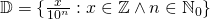. This is not quite the truth, because the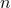actually carries information that we care about, so we would actually define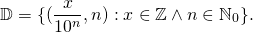So we actually want to allow the numerator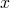to be a multiple of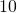and we use this to express the precision as to how many digits after the decimal point are explicitely part of our number. Having more decimal places after the decimal point expresses more precision.

Now we try to use mathematical operations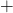,andon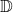. It turns out that we have three different cases. The ring operations can be defined without problems, even thoughis not quite a ring, as we will see. But it is good enough for most purposes.

•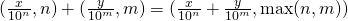•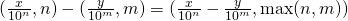•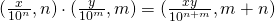Addition and Subtraction actually lose information if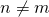, because we might have an input with lower precision and in the end pretend to have a result of the higher precision. But not losing numerical information is considered more important and implicit rounding should be avoided at all costs, at least for the basic operations.is not a ring, but it is a Semiring. The zero is not universally unique, but we seem to have many zeros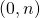. This is not the problem, because onlywould act as an additive neutral element. What we lack are additive inverse elements. If we have an elementwith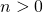, there is no element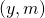, such that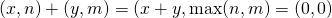. The distributivity, required for a semiring, can be seen easily:

••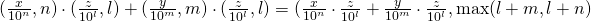But since we do computer programming and not math and only use math as a tool to help us, it is kind of OK, that it is only a semiring and not a ring, as long as we know it.

Division is a special case, because it is not always possible to express the exact numerical value of the quotient in, for example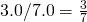, where the denominator is not a power of ten. To do such operations, a rule on how to round needs to be provided. This is cumbersome, because it blows up our formulas, so we define a set. Now the quotient of two elements ofis a member of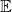. And we have the rules

•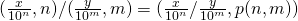••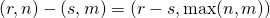•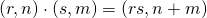•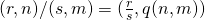where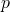and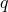somehow try to estimate how precise the result of the division might be. The basic idea is to do the whole calculation that includes the division and round the result to the desired number of decimal places after the point and with the rounding mode desired.

Now the power is a hard one. Arbitrary powers can of course be defined and are supported, but most of the time, the exponent is actually an integer. These cases can be defined nicely. For exponents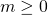we actually get a result inand for negative exponentswe get results in:

••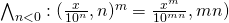For non-integral exponents, the calculation of powers falls back to Ruby’s built in power and transforms elements of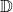andinvolved into rational numbers. These are of limited use, but they are provided and work and can be used, when needed. There is a more general power function, that has additional parameters for the desired rounding and number of digits after the decimal point. While this library goes long ways to achieve decent accuracy and speed, there are certainly possible input parameters that will result in extremely long calculation times or results that are much less accurate than claimed. Such examples are „hard“ to find and should not harm the practical usefulness of the library too much. Similar libraries in the Java world like BigDecimal do not even try to calculate powers with arbitrary exponents and the Ruby builtin library BigDecimal (which is something slightly different) does have its issues when calculating arbitrary powers.

Rounding functions are there to convert a numerical type that is at least viewable as a subset of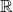to. The actual rounding has to be implemented, but it has been done for,and the built in types of Ruby except for Complex (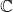). For complex numbers, the real and the imaginary part are rounded and stuffed into a new complex number.

Rounding needs two pieces of information, the desired precision (number of decimal places after the decimal point) and the rounding mode. There are different methods for rounding, but they all follow the same basic rules. A special case is the round_to_allowed_remainders, which does a residue class rounding.

There are many rounding modes. Rounding can be towards 0, away from 0, towards infinity or towards negative infinity. This boils down to cutting off all digits but(or adding zeros) and possibly adjusting the result by one, if the cut off part contained anything but zeros. Other rounding modes take a mean between the two adjacent result candidates and decide by that which one to take, requiring an extra rule for the case that the value that needs to be rounded happens to be exactly on the border.

Generalized powers and all functions that return something irrational like square roots, cubic roots, exponential functions, logarithms and in the future also trigonometric functions needs to be calculated with the number of digits required and a rounding mode. Currently square roots (sqrt) and cube roots (cbrt) are calculated accurately according to these rounding parameters. For the transcendential functions (logarithms, exponential functions, power, trigonometric functions) minor deviations from the mathematically accurate result are still possible. Since the major usage of the library is expected to deal with the basic operations only, this is considered acceptable. To really work with the transcendental functions, using interval arithmetic in conjunction with long decimal would anyway be a better way, so the necessary guarantee to be given would be to provide a result that is close, but guaranteed to be lower or equal than the real mathematical result and one that is guaranteed to be greater or equal. Progress in this area is not going to happen very soon, unless someone would be volunteering to help with this or someone would be volunteering to sponsor the development.

Also it might be interesting to port this library to other languages, even to Java, because it has become much more sophisticated than Java’s BigDecimal library. Again this is unlikely to happen too soon without any help.

The current priority is to keep this library working with recent Ruby versions and to add the missing trigonometric functions.

Use it as follows:
gem install long-decimal
to install it. Then use it in your code with:
require "long-decimal"

A remark for people who are mathematically inclined: The definition of the natural numbers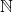is not totally universal. Sometimes we haveand sometimes we have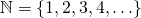. To avoid this, I am using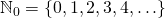, even though the indexis kind of ugly. I agree with Dijkstra that we should prefer to include the 0 in the natural numbers.
Another remark for mathematically interested readers: If we were defining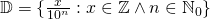, we would actually have a ring. If we now replacedwith a prime number, we would approach the realm of p-adic numbers (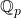). This is well worth supporting by a library as well, but it is quite a different story and of course only of interest to a small group who actually knows p-adic numbers and works with them.